Distance Formula Practice Terms of Use    Contact Person: Donna RobertsDirections: Grab a paper and pencil to make your computations.

1.
Find the length of a line segment whose endpoints are (-2,3) and (6,3).

Choose:
 4 6 8 10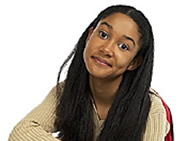2.
Find the shortest distance between the points
(-5,-3) and (2,-4).

Choose: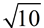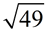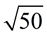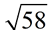3.
The point (7,2) lies on a circle. What is the length of the radius of the circle if the center is located at (2,1)?
Choose: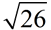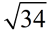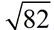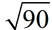4.
The endpoints of the diameter,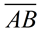, of circle O, are A(-8,-4) and B(3,6).
a) Find the center of the circle, O.
Choose:
 (2½,5) (-2½,1) (5½,1) (-5½,5)

b)
Find the length of the radius of the circle to the nearest tenth of a unit.
Choose:
 11.6 10.6 7.4 5.3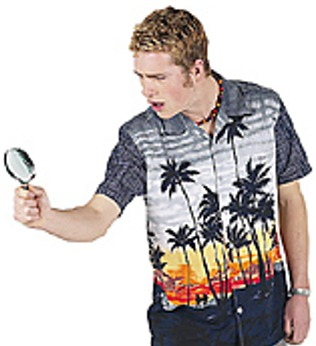5.
Given parallelogram ABCD as shown with integer coordinates. Find the length of the diagonal from A to C.

Choose: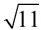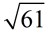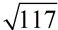15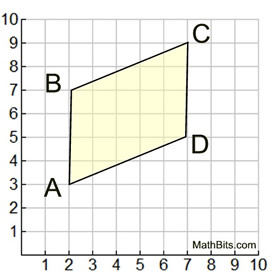6.
Given A(-1,10) and B(x,2). Find the value(s) of x if AB = 10.

Choose:
 5 only 5 or -7 6 only 6 or -67.is the diameter of a circle whose center is the point (4,-3). If the coordinates of A are
(-1,5), find AB.
Choose: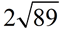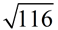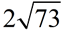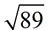8.
A chicken and a rooster, on the same straight line, are heading toward the chicken coop which is half way between them. The chicken is at A(-2,4) and the rooster is at B(4,-4).
a) What are the coordinates of the chicken coop?
Choose:
 (3, 0) (3, 4) (0, 1) (1, 0)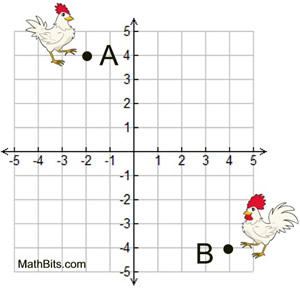b)
How far will the chicken and the rooster each have to travel to reach the chicken coop?
Choose:
 5 units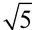units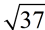units 10 units